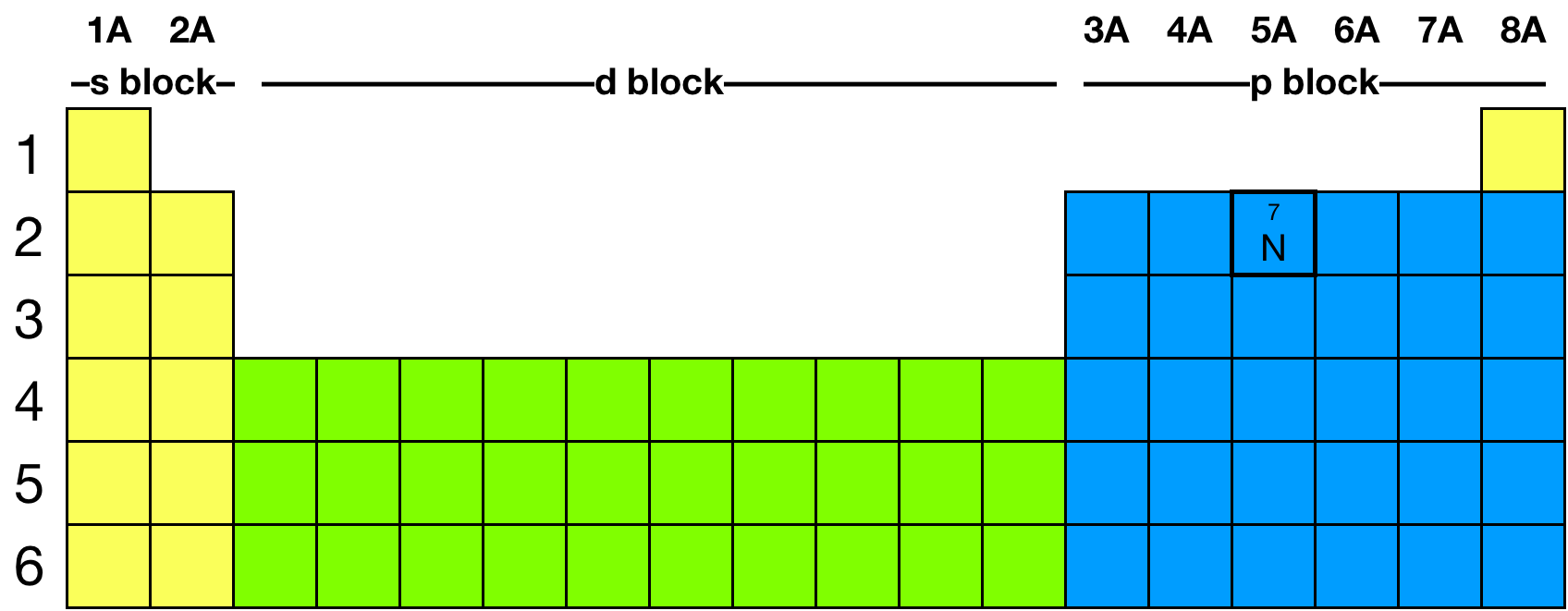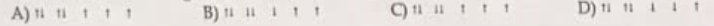# Problem: Which one of the following is the correct orbital diagram for nitrogen?

###### FREE Expert Solution

Recall that for a neutral element, Atomic number = # of protons = # of electrons

The atomic number of N is 7 which means N has 7 electrons.

The location of N in the periodic table is shown below:81% (360 ratings)###### Problem Details

Which one of the following is the correct orbital diagram for nitrogen?